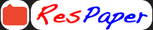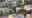Trending ▼   ResFinder# ICSE Class X Mid-term 2019 : Mathematics

5 pages, 31 questions, 0 questions with responses, 0 total responses,00Khushi Shrivastav The Future Foundation School (TFFS), Kolkata
+Fave Message
 Home > khushi_0701 >Formatting page ...

THE FUTURE FOUNDATION SCHOOL HALF YEARLY EXAMINATION SESSION 2019 2020 MATHEMATICS (Two hours and a half) (Maximum Marks : 80) CLASS X DATE 16.09.19 Answers to this Paper must be written on the paper provided separately. You will not be allowed to write during the first 15 minutes. This time is to be spent in reading the question paper. The time given at the head of this Paper is the time allowed for writing the answers. ------------------------------------------------------------------------------------------------------------Attempt all questions from Section A and a ny four questions from Section B . All working, including rough work, must be clearly shown and must be done on the same sheet as the rest of the answer. Omission of essential working will result in loss of marks. The intended marks for questions or parts of questions are given in brackets [ ]. Mathematical tables are provided. ------------------------------------------------------------------------------------------------------------------------------SECTION A (40 Marks ) ( Attempt all questions from this section ) Question 1. a)  3 + x 8x + 2 14 + 2x, x I 3 3 b) A man invested Rs.36,000 in buying Rs.100 shares at Rs.20 premium. The dividend is 15% per annum. Find i)the number of shares he buys. ii)his yearly dividend. iii)the percentage return on his investment. Give your answer correct to the nearest whole no.  c) Find the mean,median and mode of the following distribution 8,10,7,6,10,11,6,13,10  Question 2. a) Given that (x+2) and (x+3) are factors of 2x3 + ax2 + 7x b . Determine the values of a and b. b) c) Prove that tan2 (Sec 1) 2 = 1 + Cos 1 Cos The first term of an AP is 5, the last term is 45 and the sum is 400. Find the number of terms and the common difference.    This paper consists of 5 printed pages and 1 blank page. 2019 Turn overFormatting page ...Formatting page ...Formatting page ...Formatting page ...